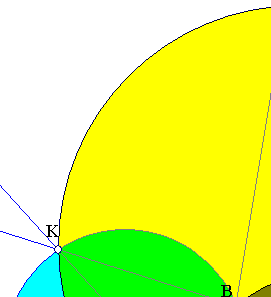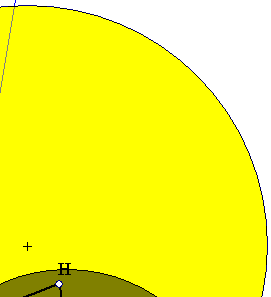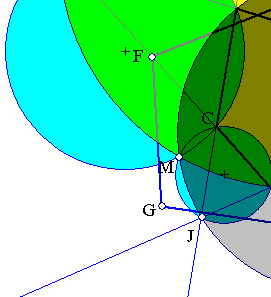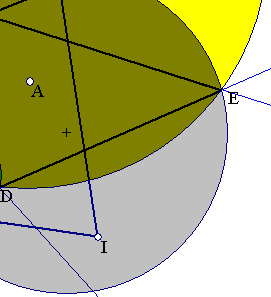##Domain of convexity for quadrangles (entire)Consider a convex quadrangle p = (BCDE) and a point A (not necessarily inside it). Build the quadrangle q = (FGIH), having as vertices the reflections of A on the sides of p. The problem is to find the domain of convexity of q. This means to find all locations for A, for which the resulting q is convex.
In the figure the convexity region is the brown region, bounded by four arcs of circles BCDEB, passing through the vertices of the quadrangle. The arcs are parts of the four circles circumscribing the four triangles formed by the prolongations of the sides of the quadrangle. When A is on one of these arcs, on BC say, then the reflections of A on the sides of triangle BCK are on a line (Steiner line passing through the corresponding orthocenter, parallel to the Simson line of A w.r. to BCK).
Look at Domain_of_Convexity_quad.html , for a related figure, concerning points A restricted inside p. The generalization for (convex) polygons with more than four sides is obvious.
By the way, when A coincides with point M, the Miquel point of the quadrangle, then q degenerates to a line segment containing the four orthocenters of the circumcircles. The problem establishes a relation of three different, seemingly unrelated, subjects: (a) Convexity, (b) Miquel points, (c) Simson lines.